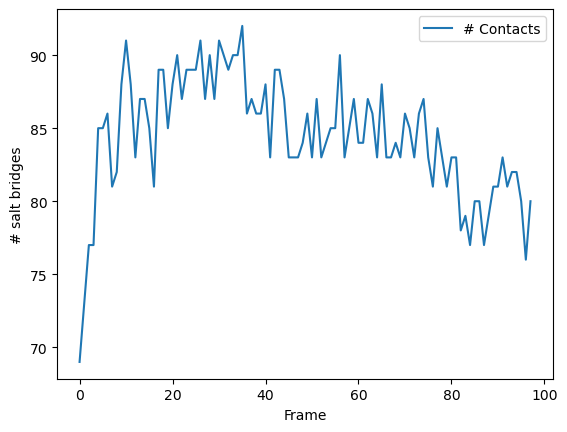# Contact analysis: number of contacts within a cutoff

We calculate the number of salt bridges in an enzyme as it transitions from a closed to an open conformation.

Last updated: December 2022 with MDAnalysis 2.4.0-dev0

Minimum version of MDAnalysis: 0.17.0

Packages required:

:

import MDAnalysis as mda
from MDAnalysis.tests.datafiles import PSF, DCD
from MDAnalysis.analysis import contacts

import numpy as np
import pandas as pd
import matplotlib.pyplot as plt
%matplotlib inline


## Background

Quantifying the number of contacts over a trajectory can give insight into the formation and rearrangements of secondary and tertiary structure. This is closely related to native contacts analysis; where the fraction of native contacts refers to the fraction of contacts retained by a protein from the contacts in a reference frame, the number of contacts simply counts how many residues are within a certain cutoff for each frame. No reference is necessary. Please see the Fraction of native contacts for an introduction to native contacts analysis.

The test files we will be working with here feature adenylate kinase (AdK), a phosophotransferase enzyme. ([BDPW09]) The trajectory DCD samples a transition from a closed to an open conformation.

:

u = mda.Universe(PSF, DCD)

/home/pbarletta/mambaforge/envs/guide/lib/python3.9/site-packages/MDAnalysis/coordinates/DCD.py:165: DeprecationWarning: DCDReader currently makes independent timesteps by copying self.ts while other readers update self.ts inplace. This behavior will be changed in 3.0 to be the same as other readers. Read more at https://github.com/MDAnalysis/mdanalysis/issues/3889 to learn if this change in behavior might affect you.


## Defining the groups for contact analysis

We define salt bridges as contacts between NH/NZ in ARG/LYS and OE*/OD* in ASP/GLU. It is not recommend to use this overly simplistic definition for real work that you want to publish.

:

sel_basic = "(resname ARG LYS) and (name NH* NZ)"
sel_acidic = "(resname ASP GLU) and (name OE* OD*)"
acidic = u.select_atoms(sel_acidic)
basic = u.select_atoms(sel_basic)


## Calculating number of contacts within a cutoff

Below, we define a function that calculates the number of contacts between group_a and group_b within the radius cutoff, for each frame in a trajectory.

:

def contacts_within_cutoff(u, group_a, group_b, radius=4.5):
timeseries = []
for ts in u.trajectory:
# calculate distances between group_a and group_b
dist = contacts.distance_array(group_a.positions, group_b.positions)
# determine which distances <= radius
timeseries.append([ts.frame, n_contacts])
return np.array(timeseries)


The results are returned as a numpy array. The first column is the frame, and the second is the number of contacts present in that frame.

:

ca = contacts_within_cutoff(u, acidic, basic, radius=4.5)
ca.shape

:

(98, 2)

:

ca_df = pd.DataFrame(ca, columns=['Frame',
'# Contacts'])

:

Frame # Contacts
0 0 69
1 1 73
2 2 77
3 3 77
4 4 85

### Plotting

:

ca_df.plot(x='Frame')
plt.ylabel('# salt bridges')

:

Text(0, 0.5, '# salt bridges')